# Flip Flop Conversion

This is the definitive guide on Flip Flop Conversion .

There are four types of flip-flops, such as:

• SR flip – flop
• D flip – flop
• JK flip – flop
• T flip – flop

Among these, the most widely used flip-flops are JK flip-flops and D flip- flops . And so their availability in the form of integrated circuits (IC’s) is abundant.

The less popular S-R flip – flop and T flip – flop are not available in the market as integrated circuits (IC’s) (even though a very few number of SR flip – flops are available as IC’s, they are not frequently used).
However, sometimes S-R and T flip-flops are also required in order to implement a logic circuit. In such cases, what we can do is to convert one type of flip-flop into another.

## Common Flip Flop Conversions

The most common types of flip-flop conversions are:

• J-K Flip-flop to S-R Flip-flop
• J-K Flip-flop to D Flip-flop
• J-K Flip-flop to T Flip-flop
• S-R Flip-flop to J-K Flip-flop
• S-R Flip-flop to D Flip-flop
• S-R Flip-flop to T Flip-flop
• D Flip-flop to S-R Flip-flop
• D Flip-flop to J-K Flip-flop

To convert one flip-flop to other type of flip-flop,  a combinational circuit is designed that is connected to the actual or available flip-flip.

Inputs to combinational circuit are same as the inputs of the required flip-flop .

Outputs of combinational circuit are connected to the inputs of the available flip-flop.

This is shown in fig.1 below :

### (i) Conversion of J-K Flip-Flop to S-R Flip-Flop

Here we will convert J-K flipflop to S-R flipflop .

So first we design a combinational circuit with S and R as its inputs and we connect its output to the input of our available flip flop i.e. an J-K flip – flop. So its outputs are same as that of S-R flip – flop.

#### step-1: Write the truth table of the Desired flip-flop

Here the desired flip-flop is S-R flip-flop. So we need to write the truth table of the S-R flip-flop in the first step.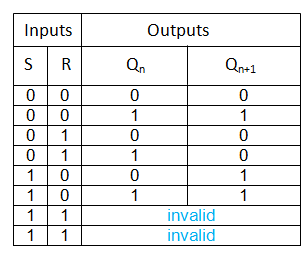#### Step 2: Obtain the Excitation Table for the given Flip-Flop from its Truth Table

What is an excitation table ??

Excitation tables provide the details regarding the inputs which must be provided to the flip-flop to obtain a definite next state (Qn+1) from the known current state (Qn). That means if we know the current state as well as the next state, we can determine the possible inputs by using the excitation table.

First we will write the truth table for J-K flip-flop as shown below.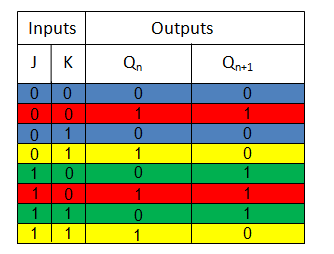Table 1: Truth Table for J-K flip-flop

From the truth table  of JK flip-flop we can see that Qn+1 will become 0 from Qn = 0 for both

(i) J = 0 and  K = 0

(ii) J = 0 and K =1 (blue entries in first and third rows of the truth table).

This means that to obtain the next state, Qn+1 as 0 from the present state Qn = 0, J must be made zero while K can be either 0 or 1.

This is indicated by the first row of the excitation table (blue entries in the first row of excitation table) where the value of K is expressed as ‘X’ indicating don’t care condition.

Similarly to obtain the next state Qn+1 as 1 from the current state Qn = 0, one has to have J equal to 1 while K can be either 0 or 1 (indicated by green entries of the truth table).

This is indicated by the second row of the excitation table (green entries in the second row of excitation table) where the value of J = 1 and K = X  ( don’t care condition) .On the same grounds, entire excitation table needs to be filled (entries in yellow  and  red colors).

#### Step 3: Obtain Conversion Table

The conversion table is obtained by filling-up the values of the J and K inputs for the given Qn and Qn+1, by referring to the excitation table.

#### Step 4: Simplify the Expressions for the Inputs of the given Flip-Flop

In this step, we will find the logical expressions for the inputs J and K in terms of S, R and Qn using suitable simplification technique like K-map .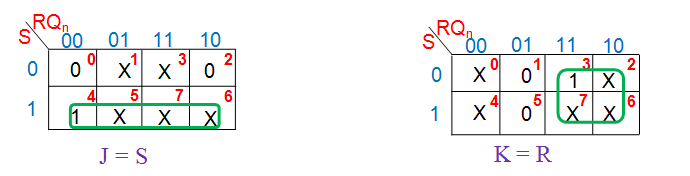#### Step 5: Design the Necessary Circuit and make the Connections accordingly

In this case no  additional circuit or new connections are needed.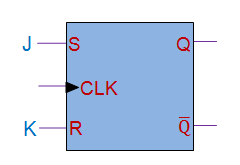Using these above steps, we can convert the given flip-flop to any other type of flip-flop .

### (ii) Conversion of JK Flip Flop to D Flip Flop

#### step-1 : Truth table for D Flip-flop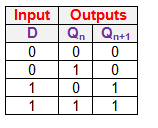#### step-2 : Excitation Table for J-K Flip-flop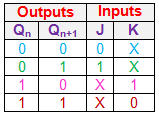#### step-3 : Conversion Table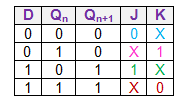#### step-4 : k-map Simplification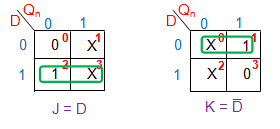#### step-5 : Circuit Design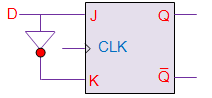### (iii) Conversion of JK Flip Flop to T Flip Flop

#### step-1 : Truth table for T Flip-flop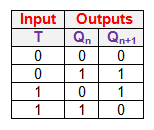#### step-2 : Excitation Table for J-K Flip-flop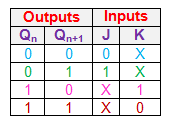#### step-3 : Conversion Table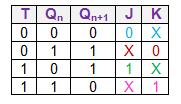#### step-4 : k-map Simplification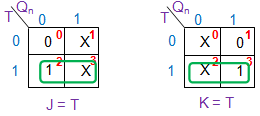#### step-5 : Circuit Design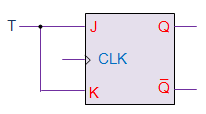### (iv) Conversion of SR Flip Flop to J-K Flip Flop

#### step-1 : Truth table for J-K Flip-flop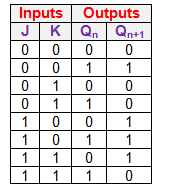#### step-2 : Excitation Table for S-R Flip-flop#### step-3 : Conversion Table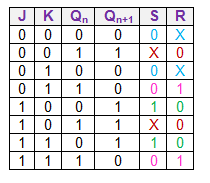#### step-4 : k-map Simplification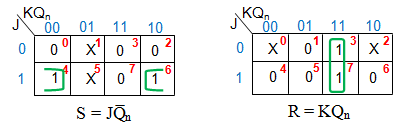#### step-5 : Circuit Design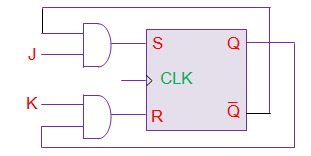### (v) Conversion of SR Flip Flop to D Flip Flop

#### step-1 : Truth table for D Flip-flop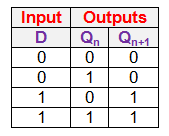#### step-2 : Excitation Table for S-R Flip-flop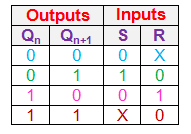#### step-3 : Conversion Table#### step-4 : k-map Simplification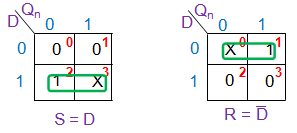#### step-5 : Circuit Design### (vi) Conversion of SR Flip Flop to T Flip Flop

#### step-1 : Truth table for T Flip-flop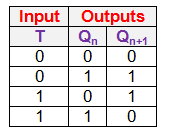#### step-2 : Excitation Table for S-R Flip-flop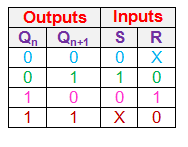#### step-3 : Conversion Table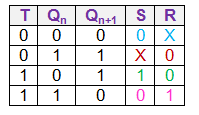#### step-4 : k-map Simplification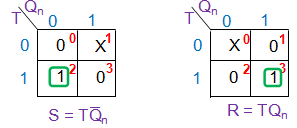#### step-5 : Circuit Design### (vii) Conversion of D Flip Flop to J-K Flip Flop

#### step-1 : Truth table for J-K Flip-flop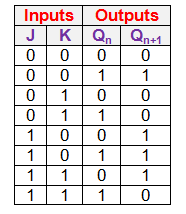#### step-2 : Excitation Table for D Flip-flop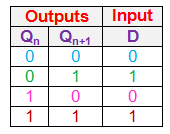#### step-3 : Conversion Table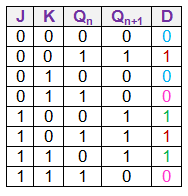#### step-4 : k-map Simplification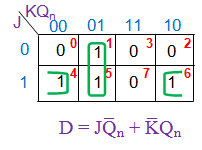#### step-5 : Circuit Design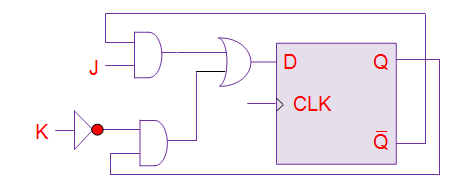### (viii) Conversion of D Flip Flop to SR Flip Flop

#### step-1 : Truth table for S-R Flip-flop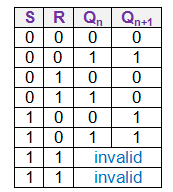#### step-2 : Excitation Table for D Flip-flop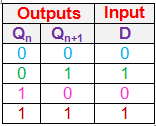#### step-3 : Conversion Table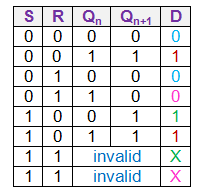#### step-4 : k-map Simplification#### step-5 : Circuit Design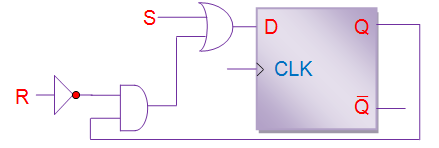### (ix) Conversion of D Flip Flop to T Flip Flop

#### step-1 : Truth table for T Flip-flop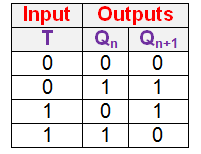#### step-2 : Excitation Table for D Flip-flop#### step-3 : Conversion Table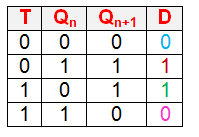#### step-4 : k-map Simplification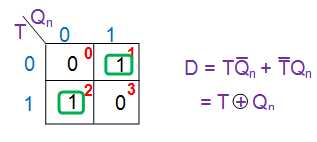#### step-5 : Circuit Design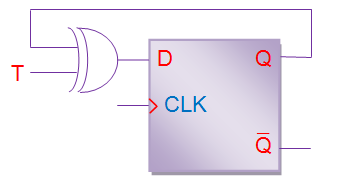### (x) Conversion of T Flip Flop to JK Flip Flop

#### step-1 : Truth table for J-K Flip-flop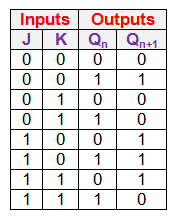#### step-2 : Excitation Table for T Flip-flop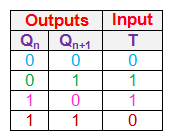#### step-3 : Conversion Table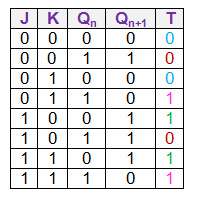#### step-4 : k-map Simplification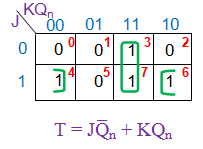#### step-5 : Circuit Design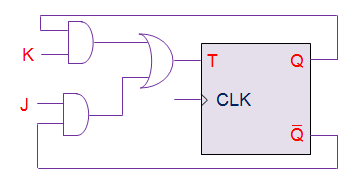### (xi) Conversion of T Flip Flop to SR Flip Flop

#### step-1 : Truth table for S-R Flip-flop#### step-2 : Excitation Table for T Flip-flop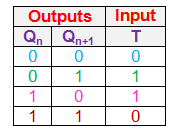#### step-3 : Conversion Table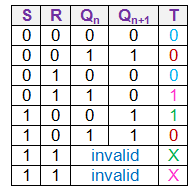#### step-4 : k-map Simplification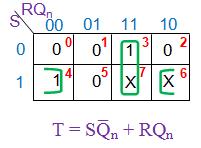#### step-5 : Circuit Design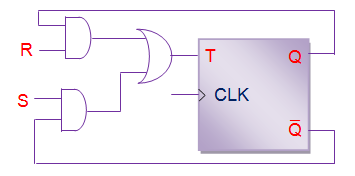### (xii) Conversion of T Flip Flop to D Flip Flop

#### step-1 : Truth table for D Flip-flop#### step-3 : Conversion Table#### step-4 : k-map Simplification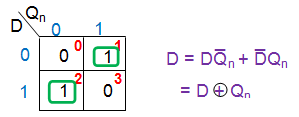#### step-5 : Circuit Design